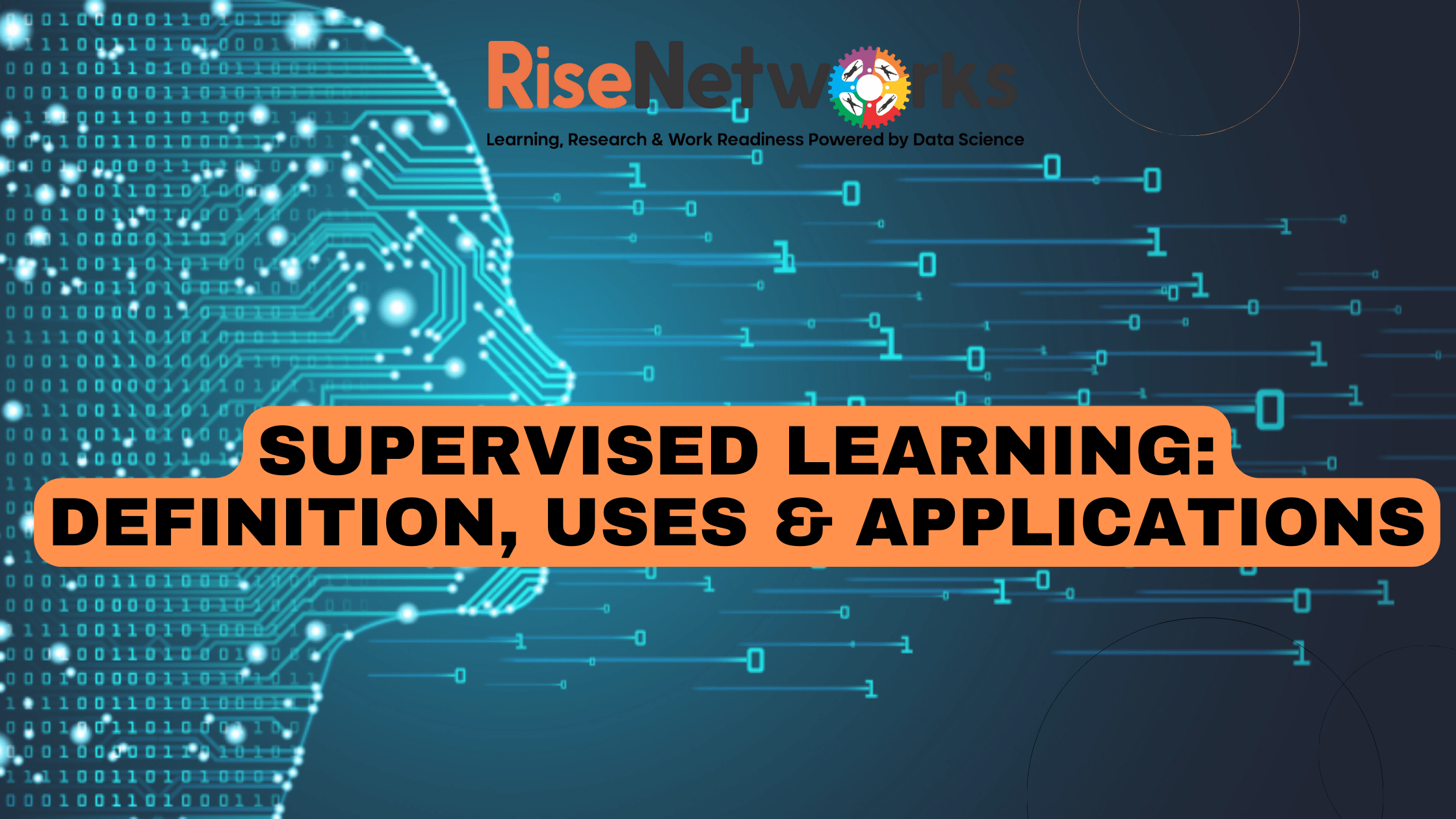Rise Networks# Supervised Learning: Definition, Uses and application

Supervised learning is a type of machine learning that utilizes labelled training data to learn the target function. Unlike unsupervised learning, which learns without any guidance or labels, supervised learning is based on training examples in which output values are known (supervision)

The term \\\”supervised\\\” refers to the fact that the algorithm is presented with a training dataset that includes the desired answers.

How supervised learning works

According to IBM supervised learning uses a training set to teach models to yield the desired output. This training dataset includes inputs and correct outputs, which allow the model to learn over time. The algorithm measures its accuracy through the loss function, adjusting until the error has been sufficiently minimized.

Types of Supervised learning

Supervised learning has two main categories: classification and regression.

Classification

Classification uses an algorithm to accurately assign test data into specific categories. It recognizes specific entities within the dataset and attempts to draw some conclusions on how those entities should be labelled or defined.

Common classification algorithms are linear classifiers, support vector machines (SVM), decision trees, k-nearest neighbours, and random forests.

Regression

Regression is used to understand the relationship between dependent and independent variables.

It is commonly used to make projections, such as for sales revenue for a given business. Linear regression, logistical regression, and polynomial regression are popular regression algorithms.

Source: IBM

Supervised learning algorithms

Supervised learning is used in applications such as:

Data mining – The process of analyzing large amounts of data to discover previously unknown patterns, trends, and associations

Computer vision – The ability of computers to interpret video images and process them in useful ways

Image recognition – The ability of computers to recognize objects in images

Natural language processing (NLP) – The development of systems that enable computers to interpret human language.

0